# A Tutorial on Multivariable Modeling

## Preliminaries

This is a short narrative of the tutorial as planned. It's main purpose to is give you a record of the computer commands in a form that can be directly executed.

### An RStudio Account

As capacity permits, you will be given an account on the RStudio server at `dahl.calvin.edu` for you to follow along. But if that's not possible, you have this document for later reference.

### The mosaic package

We've tried to create a systematic approach to the commands used in basic statistics and those used in multivariate modeling. This is distributed using the standard mechanism in R: the package.

Our package, `mosaic`, written with Randall Pruim of Calvin College, also gives a easy way to extract out the mathematical function implicit when a model is created, an easy way to graph such functions (in one and two variables), and to evaluate them to examine partial and total differences.

``````require(mosaic)
``````

## Start Simply

We're going to start by working with some data on the prices of used cars, collected from the `cars.com` site by some of our students for a course exercise. The focus of the exercise was on how the price depends on age and mileage.

``````cars = fetchData("used-hondas.csv")
``````
``````## Retrieving from http://www.mosaic-web.org/go/datasets/used-hondas.csv
``````
``````names(cars)
``````
``````##  "Price"    "Year"     "Mileage"  "Location" "Color"    "Age"
``````
``````summary(cars)
``````
``````##      Price            Year         Mileage             Location
##  Min.   : 4900   Min.   :1996   Min.   :     8   Durham    :34
##  1st Qu.:10545   1st Qu.:2001   1st Qu.: 33700   Santa Cruz:29
##  Median :14658   Median :2003   Median : 52054   St.Paul   :29
##  Mean   :14343   Mean   :2003   Mean   : 63425
##  3rd Qu.:17988   3rd Qu.:2005   3rd Qu.: 92321
##  Max.   :22995   Max.   :2007   Max.   :194500
##    Color         Age
##  Black:36   Min.   : 0.00
##  Brown:21   1st Qu.: 2.00
##  Grey :20   Median : 4.00
##  White:15   Mean   : 3.95
##             3rd Qu.: 6.00
##             Max.   :11.00
``````

It's worth pointing out a similar dataset and the associated teaching plan developed by Shonda Kuiper at Grinnell College, published online in the Journal of Statistical Education. That dataset has many other variables, but deals only with cars that are one year old.

Of course, you might want to start by looking at the `Price` data itself:

``````mean(Price, data = cars)
``````
``````##  14343
``````
``````sd(Price, data = cars)
``````
``````##  4616
``````
``````histogram(~Price, data = cars)
``````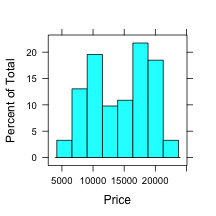You might also want to consider breaking down the price by an explanatory variable, e.g. the `Location` where the car is being offered for sale.

``````mean(Price ~ Location, data = cars)
``````
``````##     Durham Santa Cruz    St.Paul
##      14028      15340      13714
``````

Or some graphics:

``````bwplot(Price ~ Location, data = cars)
``````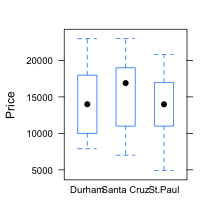``````xyplot(Price ~ Mileage, data = cars)
``````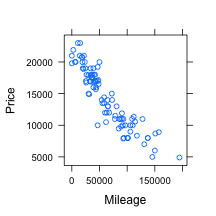Fitting a regression line to the `Price` versus `Mileage` relationship:

``````mod0 = lm(Price ~ Mileage, data = cars)
``````

This model has the standard things you associate with a model: coefficients, residuals, fitted values, etc.

``````coef(mod0)
``````
``````## (Intercept)     Mileage
##  20766.5803     -0.1013
``````

Implicit in the form of the function and the coefficients is a straight-line function that takes `Mileage` as the input. You can produce that function explicitly, name it, evaluate it. and graph it:

``````f0 = makeFun(mod0)
f0(Mileage = 50000)
``````
``````##     1
## 15702
``````
``````xyplot(Price ~ Mileage, data = cars)
plotFun(f0(Mileage) ~ Mileage, add = TRUE)
``````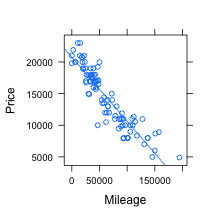You can add in more explanatory variables. For instance, perhaps the price depends on both `Mileage` and `Location`

``````mod1 = lm(Price ~ Mileage + Location, data = cars)
f1 = makeFun(mod1)
xyplot(Price ~ Mileage, data = cars)
plotFun(f1(M, Location = "Durham") ~ M, add = TRUE)
plotFun(f1(M, Location = "Santa Cruz") ~ M, add = TRUE, col = "red")
plotFun(f1(M, Location = "St.Paul") ~ M, add = TRUE, col = "green")
``````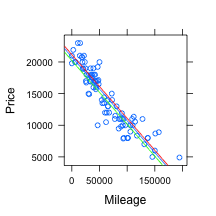It's not a coincidence that the tree lines are parallel. We didn't include an interaction term between `Mileage` and `Location` in the model. Here's another model:

``````mod2 = lm(Price ~ Mileage * Location, data = cars)
f2 = makeFun(mod2)
xyplot(Price ~ Mileage, data = cars)
plotFun(f2(M, Location = "Durham") ~ M, add = TRUE)
plotFun(f2(M, Location = "Santa Cruz") ~ M, add = TRUE, col = "red")
plotFun(f2(M, Location = "St.Paul") ~ M, add = TRUE, col = "green")
``````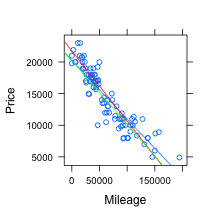Which of these models is better, `mod1` or `mod2` or, for that matter, `mod0`?

Before addressing that, let's consider a model with two quantitative explanatory variables: `Mileage` and `Price`. This is fit using the same syntax:

``````mod3 = lm(Price ~ Mileage * Age, data = cars)
``````

One way to plot out this function is to pick several ages, and make a plot of price versus mileage for each of those ages:

``````f3 = makeFun(mod3)
xyplot(Price ~ Mileage, data = cars)
plotFun(f3(M, Age = 1) ~ M, add = TRUE)
plotFun(f3(M, Age = 5) ~ M, add = TRUE, col = "red")
plotFun(f3(M, Age = 10) ~ M, add = TRUE, col = "green")
``````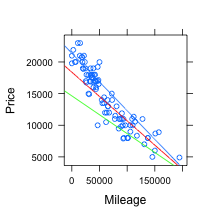Another way to make the plot uses contours to display the output, so that both input variables can be used:

``````plotFun(f3(Mileage = M, Age = A) ~ A & M, A.lim = range(0, 15), M.lim = range(0,
2e+05))
``````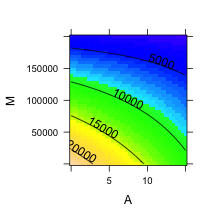We included an interaction term, just because it's easy to do. Notice that older cars see a less steep reduction in price with mileage.

#### Standard Inference Reports

We'll talk about these briefly in the breakout session. Here are examples of the commands:

``````summary(mod2)
``````
``````##
## Call:
## lm(formula = Price ~ Mileage * Location, data = cars)
##
## Residuals:
##    Min     1Q Median     3Q    Max
##  -5922  -1011     19   1069   4377
##
## Coefficients:
##                             Estimate Std. Error t value Pr(>|t|)
## (Intercept)                 2.02e+04   6.20e+02   32.66   <2e-16 ***
## Mileage                    -9.25e-02   7.89e-03  -11.72   <2e-16 ***
## LocationSanta Cruz          1.53e+03   8.75e+02    1.74    0.085 .
## LocationSt.Paul            -1.22e-01   8.81e+02    0.00    1.000
## Mileage:LocationSanta Cruz -1.86e-02   1.18e-02   -1.57    0.121
## Mileage:LocationSt.Paul    -8.32e-03   1.13e-02   -0.74    0.462
## ---
## Signif. codes:  0 '***' 0.001 '**' 0.01 '*' 0.05 '.' 0.1 ' ' 1
##
## Residual standard error: 1880 on 86 degrees of freedom
## Multiple R-squared: 0.844,   Adjusted R-squared: 0.835
## F-statistic: 93.1 on 5 and 86 DF,  p-value: <2e-16
``````
``````anova(mod2)
``````
``````## Analysis of Variance Table
##
## Response: Price
##                  Df   Sum Sq  Mean Sq F value Pr(>F)
## Mileage           1 1.62e+09 1.62e+09  459.27 <2e-16 ***
## Location          2 1.28e+07 6.39e+06    1.82   0.17
## Mileage:Location  2 8.64e+06 4.32e+06    1.23   0.30
## Residuals        86 3.02e+08 3.52e+06
## ---
## Signif. codes:  0 '***' 0.001 '**' 0.01 '*' 0.05 '.' 0.1 ' ' 1
``````

### House Prices and a Fireplace

Dick De Veaux originated this exercise and shared it with us. It concerns the prices of houses in Saratoga Springs, NY.

The particular question we want to address is the extent to which a fireplace increases the value of a house. Perhaps we fixing up a house for sale and trying to decide whether to retro-fit a fireplace into an existing house, or close off an old fireplace. What are the implications of this on the sales price of the house?

``````houses = fetchData("SaratogaHouses.csv")
``````
``````## Retrieving from http://www.mosaic-web.org/go/datasets/SaratogaHouses.csv
``````
``````names(houses)
``````
``````##  "Price"       "Living.Area" "Baths"       "Bedrooms"    "Fireplace"
##  "Acres"       "Age"
``````

The mean prices are quite different for the houses with and without a fireplace:

``````mean(Price ~ Fireplace, data = houses)
``````
``````##      N      Y
## 127955 198455
``````

Is this a useful estimate of the value of a fireplace?

Then Saratoga data to work on